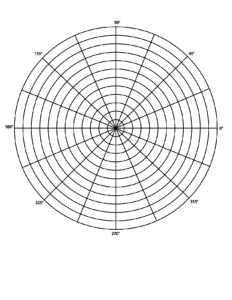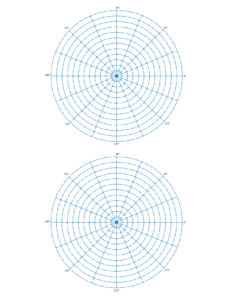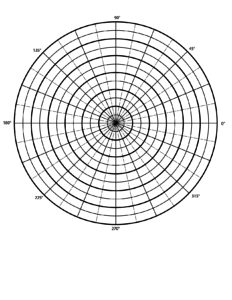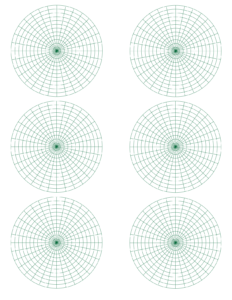Polar graph paper is a type of graph paper made up of circles with equal distance between them. All the circles are divided into small arcs by lines that show the angles of the circle (0-360).Plotting on a polar graph starts with counting out (using each circle as a guide) from the center of the pole, and then using the degree as the second coordinate.(6, 180°) is an example coordinate on a polar graph. This coordinate is a point which is 6 circles out from the center and on the 180° degree line. Because they are plotted on a circle, each point also correlates to a negative coordinate.